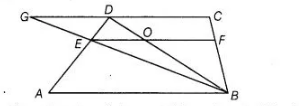# E and F are respectively the mid-points`
Question:

E and F are respectively the mid-points of the non-parallel sides AD and BC of a trapezium ABCD. Prove that EF || AB and EF = ½ (AB + CD).

Solution:

Given $A B C D$ is a trapezium in which $A B \| C D$. Also, $E$ and $F$ are respectively the mid-points of sides $A D$ and $B C$.Construction Join $B E$ and produce it to meet $C D$ produced at $G$, also draw $B D$ which intersects $E F$ at $O$.

To prove $E F \| A B$ and $E F=\frac{1}{2}(A B+C D)$.

Proof In $\Delta G C B, E$ and $F$ are respectively the mid-points of $B G$ and $B C$, then by mid-point theorem.

$E F \| G C$

But  $G C \| A B$ or $C D \| A B$ [given]

$\therefore$ $E F \| A B$

In $\triangle A D B, A B \| E O$ and $E$ is the mid-point of $A D$.

Therefore by converse of mid-point theorem, $O$ is mid-point of $B D$.

Also, $E O=\frac{1}{2} A B$ $\ldots(i)$

In $\triangle B D C, O F \| C D$ and $O$ is the mid-point of $B D$.]

$\therefore$  $O F=\frac{1}{2} C D$  [by converse of mid-point theorem] (ii)

On adding Eqs. (i) and (ii), we get

$E O+O F=\frac{1}{2} A B+\frac{1}{2} C D$

$\Longrightarrow$   $E F=\frac{1}{2}(A B+C D)$ Hence proved.